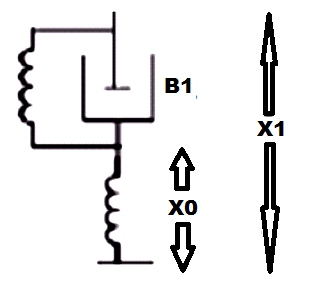# Differential Equations of Physical Systems & Dynamics of Robotic Mechanisms

Differential Equations of Physical Systems & Dynamics of Robotic Mechanisms

1. Consider a simple mass spring friction system as given in the figure K1, K2 are spring constants f-friction, M-Mass, F-Force, x-Displacement. The transfer function X(s)/F(s) of the given system will be :a) 1/(Ms2+fs+K1.K2)
b) 1/(Ms2+fs+K1+K2)
c) 1/(Ms2+fs+K1.K2/K1+K2)
d) K2/(Ms2+fs+K1)

Explanation: Force balance equations are formed where force from both the springs will be balanced by the mass system.

2. The output of an first order hold between two consecutive sampling instants is:

a) Constant
c) Ramp Function
d) Exponential Function

Explanation: Inverse Laplace of the equation of first order hold gives the ramp function and hence the output of an first order hold between two consecutive sampling is ramp function.

3. Which of the following is an example of an open loop system?

a) Household Refrigerator
b) Respiratory system of an animal
c) Stabilization of air pressure entering into the mask
d) Execution of program by computer

Explanation: Execution of a program by a computer is an example of an open loop system as the feedback mechanism is not taken by the computer program and set programs are used to get the set output.

4. A tachometer is added to servomechanism because:

c) It reduces steady state error
d) It converts velocity of the shaft to a proportional Dc voltage

Explanation: A tachometer is a device to control the speed and adjust damping and it is used in servomechanism to adjust damping and mainly is used in AC servomotors.

5. A synchro Transmitter is used with control transformer for:

a) Feedback
b) Amplification
c) Error detection
d) Remote sensing

Explanation: Synchro transmitter is used as the error detector to get the desired speed and it is accompained with the synchro transformer which is used as an amplifier.

6. The below figure represents:b) Lag network
c) PI controller
d) PD controller

Explanation: The equations of performance are
B1(dX1/dt-dX2/dt)+k1(X1-X0)=k2X0
T=k1(1+B1s/K1)/k1+k2(1+sB1/k1+k2)
X0(s)/X1(s)=1/a(1+aTs/1+Ts).

7. Backlash in a stable control system may cause:

a) Under damping
b) Over damping
c) High level oscillations
d) Low level oscillations

Explanation: In a servo system, the gear backlash may cause sustained oscillations or chattering phenomenon and the system may even turn unstable for large backlash.

8. Tachometer feedback in a D.C. position control system enhances stability?

a) True
b) False

Explanation: Tachometer feedback is derivative feedback and hence increases the stability and speed of response, so tachometer adds zero at origin.

9. For a tachometer, if a(t) is the rotor displacement, e(t) is the output voltage and K is the tachometer constant, then the transfer function is given by:

a) Ks2
b) K/s
c) Ks
d) K

Explanation: e(t)=Kw
E(s)=Ksa(s)
E(s)/a(s)=Ks.

10. Gear train in the motor is used to reduce the gear ratio?

a) True
b) False

Explanation: Gear ratio refers to the ratio of the number of teeths in the respective gears and gear train in the motor is specifically used to increase the gear ratio.

11. Assertion (A): Servomotors have heavier rotors and lower R/X ratio as compared to ordinary motors of similar ratings.
Reason (R): Servomotor should have smaller electrical and mechanical time constants for faster response.

a) Both A and R are true and R is the correct explanation of A
b) Both A and R are true but R is not correct explanation of A
c) A is true but R is false
d) A is false but R is true

Explanation: Ac servomotors are essentially induction motor with low X/R ratio for the rotor which has very low inertia.

12. Assertion (A): DC servomotors are more commonly used in armature controlled mode than field controlled mode.
Reason (R): Armature controlled Dc motors have higher starting torque than fiels controlled motors.

a) Both A and R are true and R is the correct explanation of A
b) Both A and R are true but R is not correct explanation of A
c) A is true but R is false
d) A is false but R is true

Explanation: To get higher speed in field controlled dc motor, field current is decreased with decrease in torque.

13. In case of DC servomotor, the back emf is equivalent to an “electric friction” which tends to:

a) Slowly decrease the stability of the system
b) Improve stability of the system
c) Very rapidly decrease the stability of the system
d) Have no effect of stability

Explanation: As Back emf in dc servomotors provides necessary centrifugal force to control the speed of the motor that increases the stability of the system.

14. The lagrangian is defined as:

a. Sum of kinetic energy and hydraulic energy
b. Mechanical energy
c. Difference of kinetic and potential energy
d. None of these

Explanation: By definition lagrangian is defined as difference of kinetic and potential energy.
L=K-P.

15. A gantry robot consists of a manipulator mounted on an overhead system that allows movement only in ________ plane.

a) Horizontal
b) Inclined
c) Vertical
d) None of the mentioned

Explanation: Gantry robot allows motion only in horizontal plane and this is made in this geometry to fulfill the respective work.

16. An object stationary or moving in a uniform motion w.r.t A will appear to be traveling in a straight path w.r.t B. This apparent path is attributed to Coriolis acceleration.

a) True
b) False